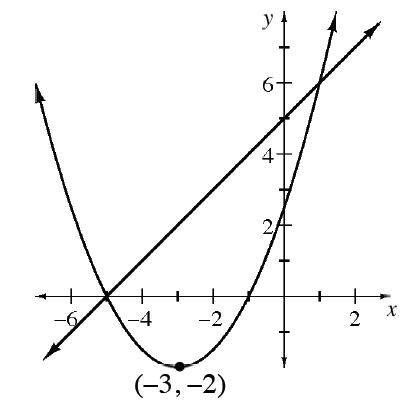### Home > INT3 > Chapter 3 > Lesson 3.2.4 > Problem3-109

3-109.

Consider the graph at right as you answer the following questions. Homework Help ✎

1. What is the equation of the parabola?

Use the vertex to help you write an equation in graphing form.

vertex: $(−3,−2)$
$y=a(x−h)^2+k$

1. What is the equation of the line?

2. Use the graph to solve $x+5=\frac{1}{2}(x+3)^2-2$. How can you verify that your solution(s) are correct?

$x=−5$ and $x=1$

3. Use the graph to solve the system below.
$y=\frac{1}{2}(x+3)^2-2$
$y=x+5$

Your answers should be in the form $(x,y)$.

4. Use the graph to solve the inequality $x+5<\frac{1}{2}(x+3)^2-2$.

$x<−5$ or $x>1$

5. Use the graph to solve $\frac{1}{2}(x+3)^2-2=0$.

$x=−5$ and $x=−1$

6. How could you change the equation of the parabola so that the parabola and the lines do not intersect? Is there more than one way?

What can you do to the equation of the parabola to change its position on the graph?
How can you move it up or flip it over?